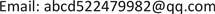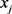﻿ 基于K-Means的时间季节反演识别方法 Time and Season Recognition Method via K-Means

Journal of Image and Signal Processing
Vol.07 No.01(2018), Article ID:23600,8 pages
10.12677/JISP.2018.71007

Time and Season Recognition Method via K-Means

Jianwen Chen, Guanlei XuReceived: Jan. 7th, 2018; accepted: Jan. 22nd, 2018; published: Jan. 30th, 2018ABSTRACT

Different time seasons have different characteristics. According to the characteristics of the season, we use various methods on the computer to clearly identify different seasons. Based on HSV color model, the characteristics of the images of each season are analyzed and studied, and in the colors contrast, the mean value and variance of them are compared. Through using the optical image processing technology, adopting the nearest neighbor classification method, comparing the characteristics of different season images, and then classifying and recognizing the selected images, thus the season can be identified automatically. The experimental results confirm the feasibility of this method, and can achieve high performance in season recognition.

Keywords:Feature, HSV Image Color Model, Neighborhood Classification, Image Identification1. 引言

2. 图像分类

2.1. 典型的分类方法

1) 神经网络分类  的方法

2) 贝叶斯分类方法

2.2. 图像聚类的研究现状及发展

1) 发现任意形状的聚类的能力

2) 输入参数对领域知识的弱依赖性

3) 初始值的选择以及输入顺序对聚类结果的影响

4) 高维数据的处理能力

3. HSV空间模型

HSV模型在1978年由埃尔维·雷·史密斯创立(图1)。

HSV色彩模型从CIE三维颜色空间演变而来，它采用的是用户直观的色彩描述方法，它跟孟塞尔显色系统的HVC球型色立体较接近。(如图HSV色彩六棱锥)只不过HSV色彩模型是一个倒立的六菱锥，只相当于孟塞尔球型色立体的一半(南半球)，所以不含黑色的纯净颜色都处于六菱锥顶面的一个色平面上  。在HSV六菱锥色彩模型中，色相(H)处于平行于六菱锥顶面的色平面上，它们围绕中心轴V旋转和变化，红、黄、绿、青、蓝、品红六个标准色分别相隔60度  。色彩明度(B)沿六菱锥中心轴V从上至下变化，中心轴顶端呈白色(V = 1)，底端呈黑色(V = 0)，它们表示无彩色系的灰度颜色。色彩饱和度(S)沿水平方向变化，越接近六菱锥中心轴的色彩，其饱和度越低，六边形正中心的色彩饱和度为零(S = 0)，与最高明度的V = 1相重合，最高饱和度的颜色则处于六边形外框的边缘线上(S = 1)  。

1) 色相、饱和度与六棱锥色平面(H和S)色平面(H、S)的基础是CIE色度图的x、y色平面。

2) 明度与六棱锥中轴色(v)色明度(V)的基础是CIE三维颜色空间的亮度因素Y。

4. 最近邻分类Figure 1. HSV model

5. K-Means聚类算法

K-means算法是硬聚类算法，是典型的基于原型的目标函数聚类方法的代表，它是数据点到原型的某种距离作为优化的目标函数，利用函数求极值的方法得到迭代运算的调整规则。K-means算法以欧式距离作为相似度，它是求对应某一初始聚类中心向量V最优分类，使得评价指标J最小。算法采用误差平方和准则函数作为聚类准则函数  。

1) 聚类个数K并不能预先确定。我们需要找到一个有效性指标，来确定最佳聚类个数。

2) 类中心点不能预先知道，需要用某种方法初设“种子”。

K-means聚类算法的主要思想可用下式描述：

$\text{Minimize}\text{ }\text{ }J\left(X,U,V\right)=\underset{i=1}{\overset{k}{\sum }}\underset{j=1}{\overset{n}{\sum }}{u}_{ij}{‖{x}_{j}-{v}_{i}‖}^{2}$ (1)

$X=\left\{{x}_{1},{x}_{2},\cdots ,{x}_{n}\right\}\subset {R}^{S}$ 是给定的数据集， $V=\left\{{v}_{1},{v}_{2},\cdots ,{v}_{k}\right\}\subset {R}^{S}$ 是类中心点，用 ${X}_{1},{X}_{2},\cdots ,{X}_{k}$ 表示k个类， ${n}_{i}$ 表示 ${X}_{i}$ 中数据个数， $U={\left({u}_{ij}\right)}_{k×n}$ 是聚类矩阵，由${X}_{i}$ 的成员关系组成， $d\left(x,y\right)=‖x-y‖,x,y\in {R}^{S}$ 是一个距离函数(例如，欧几里得距离)。为了极小化 $J\left(X,U,V\right)$ 类中心点 ${v}_{i}\left(i=1,2,\dots ,k\right)$ 和成员关系矩阵 $U$ 需要用以下的迭代公式逐步计算：

${u}_{ij}=\left\{\begin{array}{l}1;\text{\hspace{0.17em}}\text{\hspace{0.17em}}‖{x}_{j}-{v}_{i}‖\le ‖{x}_{j}-{v}_{h}‖,h=1,2,\dots ,k,\text{\hspace{0.17em}}h\ne i\\ 0;\text{\hspace{0.17em}}\text{\hspace{0.17em}}否则\end{array}$ (2)

${v}_{i}=\frac{\underset{j=1}{\overset{n}{\sum }}{u}_{ij}{x}_{j}}{\underset{j=1}{\overset{n}{\sum }}{u}_{ij}}=\frac{\underset{{x}_{j}\in {X}_{i}}{\sum }{x}_{j}}{{n}_{i}}$ (3)

${u}_{ij}\left(i=1,2,\dots ,k,j=1,2,\dots ,n\right)$ 。K-means算法就是基于(2)、(3)的迭代过程： ${V}_{t-1}\to {U}_{t}\to {V}_{t}$ ，直到 $‖{v}_{t}-{v}_{t-1}‖\le \epsilon$ 。其中 $\epsilon$ 是给定终止条件。最终的聚类结果通过成员关系矩阵确定，即如果 ${u}_{ij}=1$ ，则说明 ${x}_{ij}\left(j=1,2,\dots ,n\right)$ 属于类 ${X}_{i}\left(1\le i\le k\right)$  。

1) 输入聚类数口 $k$ ，确定距离函数，给定迭代终止条件 $\epsilon$

2) 初始化中心点谓 ${v}_{i}^{0}\left(i=1,2,\cdots ,k\right)$

3) 利用方程式(2)计算 ${u}_{ij}\left(i=1,2,\cdots ,k,\text{\hspace{0.17em}}j=1,2,\cdots ,n\right)$

4) 利用方程式(3)计算新的中心点 ${v}_{i}^{1}\left(i=1,2,\cdots ,k\right)$

5) 如果 $\underset{1\le i\le k}{\mathrm{max}}‖{v}_{i}^{0}-{v}_{i}^{1}‖\le \epsilon$ ，那么迭代终止，转向6)，否则令： ${v}_{i}^{0}={v}_{i}^{1}\text{\hspace{0.17em}}\left(i=1,2,\cdots ,k\right)$ 转向3)；

6) 出分类结果：类中心点 ${v}_{i}^{1}\left(i=1,2,\cdots ,k\right)$ 和成员关系矩阵 $U$

7) 终止。

1) 选取 ${k}_{\mathrm{min}}$${k}_{\mathrm{max}}$

2) For $k={k}_{\mathrm{min}}$ to ${k}_{\mathrm{max}}$ ;

① 初始化类中心点；

② 利用算法A，更新计算 ${U}^{k}$${V}^{k}$

③ 检查终止条件，如不满足，则转向②；

④ 利用方程式(4)计算 ${V}_{km}\left(k\right)$ ，转向2)；

3) 选择 ${k}_{opt}$ 使聚类有效函数 ${V}_{km}\left(k\right)$ 达到最优(最小)；

4) 输出聚类结果：类中心点砂 ${V}_{{k}_{opt}}$ ，成员关系矩阵 ${U}_{{k}_{opt}}$ ，最佳聚类数目 ${k}_{opt}$

5) 终止。

5.1. 利用HSV空间模型进行特征提取

5.2. K-Means聚类法的实现

5.3. K-NN最邻近法的实现

6. 总结Table 2. The seasonal tonal feature data of clustering algorithm

Time and Season Recognition Method via K-Means[J]. 图像与信号处理, 2018, 07(01): 57-64. http://dx.doi.org/10.12677/JISP.2018.71007

1. 1. Worf, W. (1996) Key Frame Selection by Motion Analysis. 1996 IEEE International Conference on Acoustics, Speech, and Signal Processing, Atlanta, 9 May 1996, 1228-1231.

2. 2. Zhang, H.J., Wu, J.H., Zhang, D., et al. (1997) An Integrated System for Con-tent-based Video Retrieval and Browsing. Pattern Recognition, 30, 643-658. https://doi.org/10.1016/S0031-3203(96)00109-4

3. 3. Han, J., Kamber, M. and Pei, J. (2006) Data mining: Concepts and Techniques. Morgan Kaufmann, Burlington, Massachusetts.

4. 4. 滕敏, 卫文学, 滕宁. K-最近邻分类算法应用研究[J]. 软件导刊, 2015(3): 44-46.

5. 5. [美]冈萨雷斯, [美]伍兹. 数字图像处理[M]. 第3版. 阮秋琦, 译. 北京: 电子工业出版社, 2001.

6. 6. 张彩华. 基于颜色和纹理特征的图像检索[D]: [硕士学位论文]. 哈尔滨: 哈尔滨理工大学, 2010.

7. 7. 顾宁. 基于颜色和纹理特征的图像检索算法研究[D]: [硕士学位论文]. 南京: 南京邮电大学, 2011.

8. 8. 葛静祥. 图像纹理特征提取及分类算法研究[D]: [硕士学位论文]. 天津: 天津大学, 2010.

9. 9. 王惠明, 史萍. 图像纹理特征的提取方法[J]. 中国传媒大学学报(自然科学版), 2006, 13(1): 49-52.

10. 10. 幸锐. 基于纹理的图像聚类研究[D]: [博士学位论文]. 杭州: 浙江大学, 2009.

11. 11. 谢菲. 图像纹理特征的提取和图像分类系统研究及实现[D]: [硕士学位论文]. 成都: 电子科技大学, 2009.

12. 12. 蒋良孝. 朴素贝叶斯分类器及其改进算法研究[D]: [博士学位论文]. 武汉: 中国地质大学, 2009.

13. 13. 沈国杰. 一种基于模糊关联分类的遥感图像分类方法[J]. 计算机研究与发展, 2012, 49(7): 1500-1506.

14. 14. 肖靓. 基于支持向量机的图像分类研究[D]: [硕士学位论文]. 上海: 同济大学, 2006.

15. 15. 云峰, 周玲, 于俊清, 徐涛, 管涛. 基于局部特征聚合的图像索引方法[J]. 计算机学报, 34(11): 2224-2233.

16. 16. 陈慧. 基于内容的图像检索技术研究[J]. 福建金融管理干部学院学报, 2007(6): 55-59.

17. 17. 刘燕, 邝颖杰. 基于混合索引的图像检索系统的设计与实现[J]. 农业网络信息, 2007(6): 34-36.

18. 18. 魏志静. 基于人工神经网络的分类方法研究及其在个人信用评估中的应用[D]: [硕士学位论文]. 山东师范大学, 2007.

19. 19. 孙秀亮. 基于属性加权的选择性朴素贝叶斯分类研究[D]: [硕士学位论文]. 哈尔滨: 哈尔滨工程大学, 2013.

20. 20. 王丹, 吴孟达. 粗糙模糊C-均值算法及其在图像聚类中的应用[J]. 国防科技大学学报, 2007, 29(2): 76-80.